Chapters

Below is a 13 minute lesson from your tube on the basic math application of right triangle trig.

Link for the video above,http://youtu.be/nBwG3SXdl6E

Here is a link to a slide show from the video in case you want to look at something further. Click here.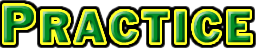• Problems
• Top Row's Solutions
• Bottom Row's Solutions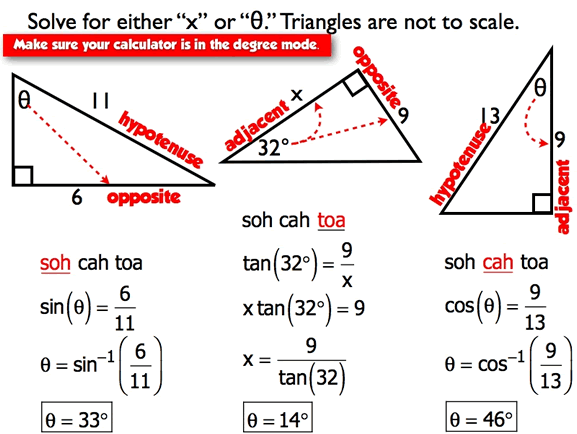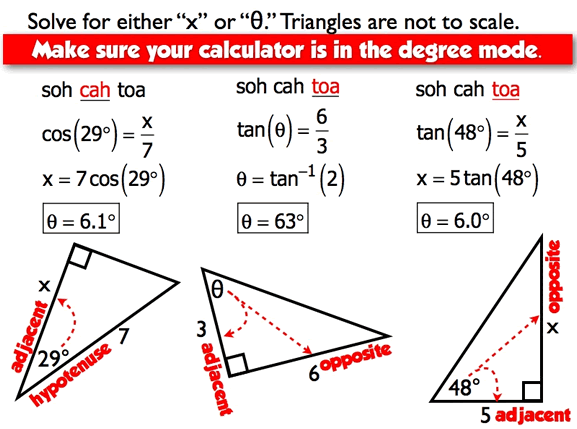Find the length of the side labeled with an "x."Answer sin25=3/x 7.10 Answer tan35=x/8 5.60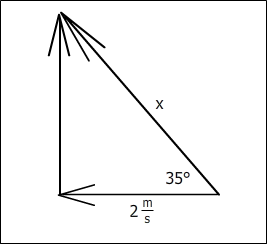Answer cos35=2/x 2.44 Answer cos35=x/11 x=9.01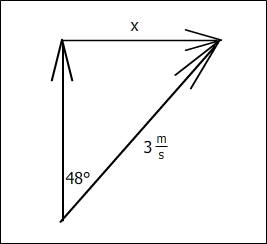Answer tan65=25/x 11.66 Answer sin48=x/3 x=2.23Answer tan73=8/x x=2.45 Answer sin62=4/x x=4.53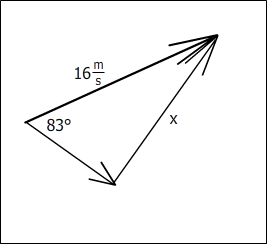Answer sin83=x/16 x=15.88 Answer tan68=x/2 x=4.95 Find the measure of the angle identified with the Greek letter θ. This symbol, θ , is the called "theta." Make sure your calculator is the degree mode.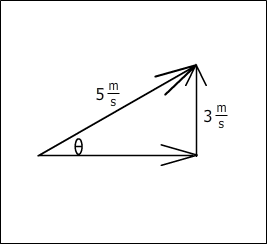Answer tan(x)=3/10 x=16.70 Answer sin(x)=3/5 x=36.87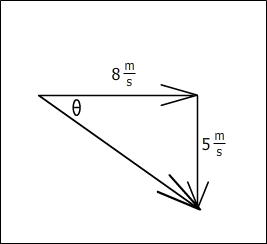Answer tan(x)=5/8 x=32.01 Answer cos(x)=9/11 x=35.10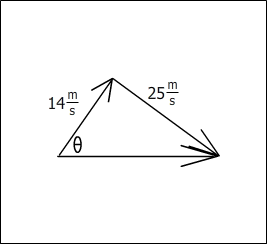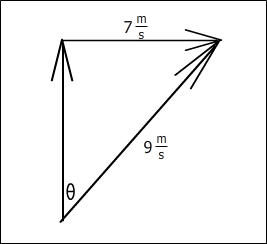Answer tan(x) = 25/14 x=60.75 Answer sin(x) = 7/9 x = 51.6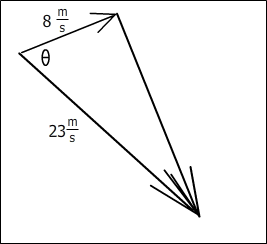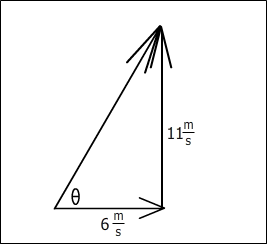Answer cos(x) = 8/23 x = 69.65 Answer tan(x) = 11/6 x = 61.39Answer tan(x) = 3/1 x = 71.57 Answer sin(x) = 2/3 x = 41.81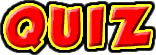This is a short right triangle trig review quiz.

Answers to worksheet "Sheet C."

by Tony Wayne ...(If you are a teacher, please feel free to use these resources in your teaching.)